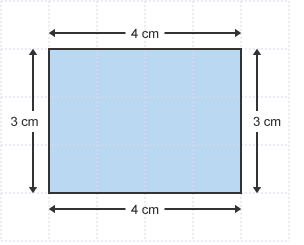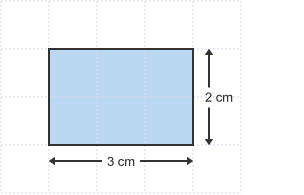# Maths Support Autumn 2 Week 2

Next week, the children will be looking at multiplication and division in maths. They will be:

-Multiplying and dividing numbers mentally drawing upon known facts.

-Know and use the vocabulary of prime numbers, prime factors and composite (non-prime) numbers

-Establish whether a number up to 100 is prime and recall prime numbers up to 19.

What are prime numbers?

https://www.bbc.co.uk/education/clips/zytrk7h

An introduction to prime numbers, composite numbers and factors. We learn that the number 1 is neither prime nor composite, and the idea of factor pairs is introduced. We look at how to decide whether a natural number is prime or composite by looking for proper factors.

Multiplying and Dividing

The children will multiply 2-digit numbers by x2 to x12, using the following method: 16 x 7 = (10 x 7 = 70)  + (6 x 7 = 42) = 70 + 42 = 112.

They will also divide 3-digit numbers by x2 to x12, using the following method: 147 ÷ 7  = (140 ÷ 7 = 20) + (7÷ 7 = 1) = 21

# Vikings Autumn 2

Hi folks,

Next term in Year 5 , we will be focusing on the viscous Vikings! This will include: where they are from, what made the come to Britain, as well as the history and background of the Vikings.

Before we do that, Miss Mitchell and I would like to know what you guys know already about the Vikings.  This could include anything from above. It doesn’t have to be long, just a few points about them.

Have a fun, safe half term and well done on all your hard efforts this term.

Year 5 Team

# Maths Support Autumn 2 Week 1

After half term, the children will be looking at factors and multiples in maths. They will be identifying  multiples and factors including finding all factor pairs of a number and common factors of two numbers.

# Factors

Factors are numbers that divide exactly into another number.

The factors of 12, for example, are 1, 2, 3, 4, 6 and 12.

Factors can be shown in pairs. The factors of 12 can be shown:

• 1 and 12 1 x 12 = 12
• 2 and 6 2 x 6 = 12
• 3 and 4 3 x 4 = 12

Each pair multiplies to make 12.

# Multiples

Multiples are really just extended times tables.

• The multiples of 2 are all the numbers in the 2 times table:
2, 4, 6, 8, 10 and so on.
Multiples of 2 always end with a 2, 4, 6, 8 or 0. You can tell 2286, for example, is a multiple of 2 because it ends with a 6.
• The multiples of 5 are all the numbers in the 5 times table:
5, 10, 15, 20, 25 and so on.
Multiples of 5 always end with a 5 or a 0. You can tell 465, for example, is a multiple of 5 because it ends with a 5.

As part of our reflection task this half term, the children have created a game from Scratch related to the rainforest. The children worked in pairs to design a background, sprite and script to showcase in their game. They also used sound, questions and trap doors to further enhance their game. Overall, the children demonstrated a fantastic level of computing skills and knowledge in creating their chosen game.

As we are coming to the end of this term, Miss Mitchell and I thought it would be nice for you guys to write a small review about what you have learnt so far during this terms topic. It could be anything to do with the rainforest, life cycles of animals or even a brief book review on the Jungle book itself.

We can not wait to hear from you guys!

# Maths Support Week 6 Autumn 1

Next week the children will be focussing on addition and subtraction. They will be adding and subtracting whole numbers using formal written methods.

Writing it down

When writing down sums, separate the numbers into ones, tens, hundreds and thousands. List the numbers in a column and always start adding with the units first.

So when adding together 7948 + 1223, you should write it down like this:• Estimate first and check afterwards – it’s a good idea to estimate a rough answer first and then check your actual answer.
• Order doesn’t matter – remember that in addition 394 + 88 is the same as 88 + 394.
• Key words – look out for these words in problems: total, sum, altogether, more. They all indicate an addition calculation.

## Writing it down

If the numbers are too high or too difficult to subtract in your head, write them down in columns. Always start subtracting with the ones first. If the digit on top is smaller than the bottom digit then we simply exchange from the column to the left. See example below.# Maths Support Autumn 1 Week 5

Next week in maths, the children will be covering measurements (in particular area and perimeter). The children will measure and calculate the perimeter of composite rectilinear shapes in cm and m. They will also look at calculating & comparing the area of rectangles (including squares), & including using standard units, square centimetres (cm2) and square metres (m2) & estimate the area of irregular shapes.

The perimeter of a shape is the distance around its boundary. This is a length, and is said to have 1 dimension.

## Example

An ant walks all the way around the edge of a rectangle, measuringby.

What distance will it have travelled?PerimeterHow to find the area of a shape?

When we find an area of a shape, we simply multiply the width by the height. For example, in the rectangle below we simply multiply the height (2cm) by the width (3cm), which gives us an answer of 6cm squared or 6cm2# Knowsley Safari Park Trip

As part of our rainforest topic this term, the children visited Knowsley Safari Park to gain further knowledge and understanding on the different layers in the rainforest, as well as the animals that live there. During the trip, the children were treated to a rainforest workshop, were they learnt about the different layers and climate in the jungle. They also came close to some animals that inhabit the different layers.

As well as a workshop, the children were taken on a guided tour of the safari park, were they encounted a wide range of different animals, including: tigers, monkeys, deer, bisens and camels. Overall, the children had an amazing time and definitely took a lot away with them from the trip.